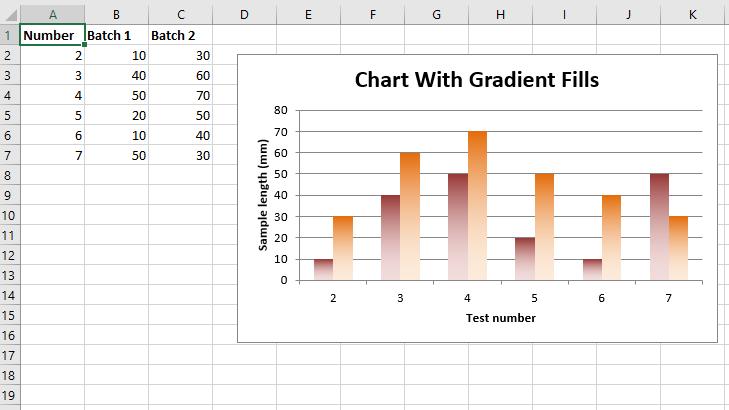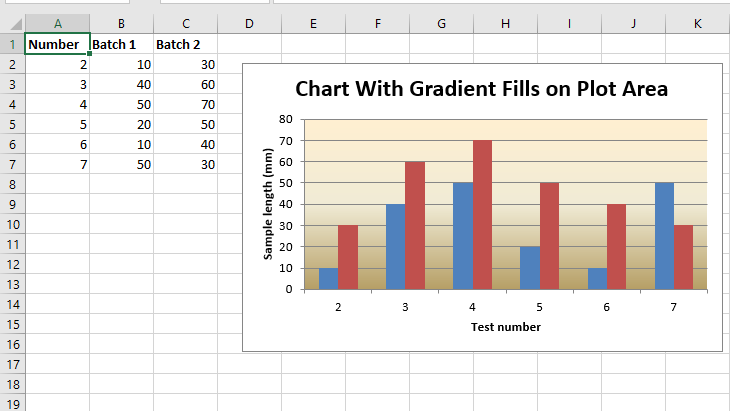# Python | Plotting an Excel chart with Gradient fills using XlsxWriter module

• Difficulty Level : Medium
• Last Updated : 30 Jan, 2022

Prerequisite: Create and Write on an excel sheet
XlsxWriter is a Python library using which one can perform multiple operations on excel files like creating, writing, arithmetic operations, and plotting graphs. Let’s see how to plot a chart with Gradient fills, using real-time data.
Charts are composed of at least one series of one or more data points. Series themselves are comprised of references to cell ranges. For plotting the charts on an excel sheet, firstly, create a chart object of a specific chart type( i.e Column chart, etc.). After creating chart objects, insert data in them, and lastly, add that chart object in the sheet object.

Code #1: Plot a Chart with Gradient fills in columns.
For plotting this type of chart on an excel sheet, use add_series() method with ‘gradient’ keyword argument of the chart object.

## Python3

 `# import xlsxwriter module``import` `xlsxwriter` `# Workbook() takes one, non-optional, argument  ``# which is the filename that we want to create.``workbook ``=` `xlsxwriter.Workbook(``'chart_gradient1.xlsx'``)` `# The workbook object is then used to add new  ``# worksheet via the add_worksheet() method.``worksheet ``=` `workbook.add_worksheet()` `# Create a new Format object to formats cells``# in worksheets using add_format() method .``  ` `# here we create bold format object .``bold ``=` `workbook.add_format({``'bold'``: ``1``})` `# Add the worksheet data that the charts will refer to.``headings ``=` `[``'Number'``, ``'Batch 1'``, ``'Batch 2'``]``data ``=` `[``    ``[``2``, ``3``, ``4``, ``5``, ``6``, ``7``],``    ``[``10``, ``40``, ``50``, ``20``, ``10``, ``50``],``    ``[``30``, ``60``, ``70``, ``50``, ``40``, ``30``],``]`  `# Write a row of data starting from 'A1'``# with bold format``worksheet.write_row(``'A1'``, headings, bold)` `# Write a column of data starting from ``# 'A2', 'B2', 'C2' respectively .``worksheet.write_column(``'A2'``, data[``0``])``worksheet.write_column(``'B2'``, data[``1``])``worksheet.write_column(``'C2'``, data[``2``])` `# Create a chart object that can be added``# to a worksheet using add_chart() method.` `# here we create a column chart object``chart ``=` `workbook.add_chart({``'type'``: ``'column'``})` `# Add a data series with Gradient to a chart``# using add_series method.``  ` `# Configure the first series .``# = Sheet1 !\$A\$1 is equivalent to ['Sheet1', 0, 0].` `# note : spaces is not inserted in b / w``# = and Sheet1, Sheet1 and !``# if space is inserted it throws warning.``chart.add_series({``    ``'name'``:       ``'= Sheet1 !\$B\$1'``,``    ``'categories'``: ``'= Sheet1 !\$A\$2:\$A\$7'``,``    ``'values'``:     ``'= Sheet1 !\$B\$2:\$B\$7'``,``    ``'gradient'``:   {``'colors'``: [``'# 963735'``, ``'# F1DCDB'``]}``})` `# Configure the second series, including a gradient.``chart.add_series({``    ``'name'``:       ``'= Sheet1 !\$C\$1'``,``    ``'categories'``: ``'= Sheet1 !\$A\$2:\$A\$7'``,``    ``'values'``:     ``'= Sheet1 !\$C\$2:\$C\$7'``,``    ``'gradient'``:   {``'colors'``: [``'# E36C0A'``, ``'# FCEADA'``]}``})` `# Add a chart title``chart.set_title ({``'name'``: ``'Chart With Gradient Fills'``})` `# Add x-axis label``chart.set_x_axis({``'name'``: ``'Test number'``})` `# Add y-axis label``chart.set_y_axis({``'name'``: ``'Sample length (mm)'``})` `# Turn off the chart legend.``chart.set_legend({``'none'``: ``True``})` `# add chart to the worksheet with given``# offset values at the top-left corner of``# a chart is anchored to cell D2 .``worksheet.insert_chart(``'D2'``, chart,``  ``{``'x_offset'``: ``25``, ``'y_offset'``: ``10``})` `# Finally, close the Excel file ``# via the close() method. ``workbook.close()`

Output :Code #2: Plot a Chart with Gradient fills in the plot area.
For plotting this type of chart on an excel sheet, use set_plotarea() method with ‘gradient’ keyword argument of the chart object.

## Python3

 `# import xlsxwriter module``import` `xlsxwriter` `# Workbook() takes one, non-optional, argument  ``# which is the filename that we want to create.``workbook ``=` `xlsxwriter.Workbook(``'chart_gradient2.xlsx'``)` `# The workbook object is then used to add new  ``# worksheet via the add_worksheet() method.``worksheet ``=` `workbook.add_worksheet()` `# Create a new Format object to formats cells``# in worksheets using add_format() method .``  ` `# here we create bold format object .``bold ``=` `workbook.add_format({``'bold'``: ``1``})` `# Add the worksheet data that the charts will refer to.``headings ``=` `[``'Number'``, ``'Batch 1'``, ``'Batch 2'``]``data ``=` `[``    ``[``2``, ``3``, ``4``, ``5``, ``6``, ``7``],``    ``[``10``, ``40``, ``50``, ``20``, ``10``, ``50``],``    ``[``30``, ``60``, ``70``, ``50``, ``40``, ``30``],]`  `# Write a row of data starting from 'A1'``# with bold format``worksheet.write_row(``'A1'``, headings, bold)` `# Write a column of data starting from ``# 'A2', 'B2', 'C2' respectively .``worksheet.write_column(``'A2'``, data[``0``])``worksheet.write_column(``'B2'``, data[``1``])``worksheet.write_column(``'C2'``, data[``2``])` `# Create a chart object that can be added``# to a worksheet using add_chart() method.` `# here we create a column chart object``chart ``=` `workbook.add_chart({``'type'``: ``'column'``})` `# Add a data series to a chart``# using add_series method.``  ` `# Configure the first series .``# = Sheet1 !\$A\$1 is equivalent to ['Sheet1', 0, 0].` `# note : spaces is not inserted in b / w``# = and Sheet1, Sheet1 and !``# if space is inserted it throws warning.``chart.add_series({``    ``'name'``:       ``'= Sheet1 !\$B\$1'``,``    ``'categories'``: ``'= Sheet1 !\$A\$2:\$A\$7'``,``    ``'values'``:     ``'= Sheet1 !\$B\$2:\$B\$7'``,})` `# Configure the second series.``chart.add_series({``    ``'name'``:       ``'= Sheet1 !\$C\$1'``,``    ``'categories'``: ``'= Sheet1 !\$A\$2:\$A\$7'``,``    ``'values'``:     ``'= Sheet1 !\$C\$2:\$C\$7'``,})` `# Set a gradient for the plotarea.``chart.set_plotarea({``    ``'gradient'``: {``'colors'``: [``'# FFEFD1'``, ``'# F0EBD5'``, ``'# B69F66'``]}``})`  `# Add a chart title``chart.set_title ({``'name'``: ``'Chart With Gradient Fills on Plot Area'``})` `# Add x-axis label``chart.set_x_axis({``'name'``: ``'Test number'``})` `# Add y-axis label``chart.set_y_axis({``'name'``: ``'Sample length (mm)'``})` `# Turn off the chart legend.``chart.set_legend({``'none'``: ``True``})` `# add chart to the worksheet with given``# offset values at the top-left corner of``# a chart is anchored to cell D2 .``worksheet.insert_chart(``'D2'``, chart,``   ``{``'x_offset'``: ``25``, ``'y_offset'``: ``10``})` `# Finally, close the Excel file ``# via the close() method. ``workbook.close()`

Output :My Personal Notes arrow_drop_up